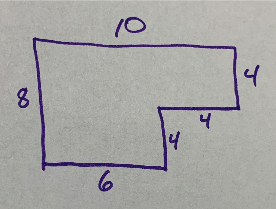100
Is 73 even or odd?
odd
100
What is the next number in the following number pattern: 30,40,50,60,70,80, ___
What is 90
100

Find the product of 3 X 4

12

100

64

100

7 O 3

A) >

B) <

>

200
Is 198 even or odd
even
200

What is the value of the 8 in 82

80 (eight 10s)

200

Find the product of 7 X 2

14

200

Subtract mentally: 49-40

9

200

18 O 20

A) >

B) <

<

300

An odd number and an even number are added together. Will their sum always be even or odd?

odd

300

What is perimeter and is it measured in "units" or "square units"?

Perimeter is the distance AROUND THE OUTSIDE  of a shape. It is measured in "units" (feet, meters, etc.)

300

36 ÷ 6 = ___

6

300

5 X __ = 25

5

300

10 X 2 __ 2 X 10

> = <

=

400

Two odd numbers are added together. Will the sum always be even or odd?

even

400

What is area and is it measured in "units" or "square units"?

Area is the space INSIDE of a shape. It is measured in "square units" (square feet, square meters, etc.)

400

45 ÷ 5 = ___

9

400

What is the next number in the pattern

36, 30, 24,....

18

400

56-24 __ 8 X 3

> = <

>

500
What is the next number in the number pattern: 33,37,41,45, ___
What is 49
500What is the area of this figure (measured in FEET)?

64 square feet

500

110 ÷ 11 = ___

10

500

Is the Numerator on the top of a fraction or the bottom? What information does it share with us?

Top - it tells us how many parts of the whole we are using.

500

Is the denominator on the top of the fraction or the bottom? What information does it share with us?

Bottom - it tells us how many parts it takes to make a whole.

Click to zoom